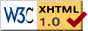# CAOSP abstracts, Volume: 45, No.: 1, year: 2015

• Author(s): Zeraatgari, F.Z.; Abedi, A.; Farshad, M.; Ebadian, M.; Riazi, N.
• Journal: Contributions of the Astronomical Observatory Skalnaté Pleso, vol. 45, no. 1, p. 5-16.
• Date: 4/2015
• Title: Neural network analysis of W UMa eclipsing binaries
• Keyword(s): Neural Network, data analysis, eclipsing binary, W UMa sytems
• Pages: 5 -- 16

Abstract: We try five different artificial neural models, four models based on PNN (Perceptron Neural Network), and one using GRNN (Generalized Regression Neural Network) as tools for the automated light curve analysis of W UMa-type eclipsing binary systems. These algorithms, which are inspired by the Rucinski method, are designed and trained using MATLAB 7.6. A total of 17,820 generated contact binary light curves are first analyzed using a truncated cosine series with 11 coefficients and the most significant coefficients are applied as inputs of the neural models. The required sample light curves are systematically generated, using the WD2007 program (Wilson and Devinney 2007). The trained neural models are then applied to estimate the geometrical parameters of seven W UMa-type systems. The efficiency of different neural network models are then evaluated and compared to find the most efficient one.

Full text version of this article in PostScript (600dpi) format compressed by gzip; or in PDF.

Back to:
CAOSP Vol. 45 No. 1 index
CAOSP archive main index
CAOSP main page